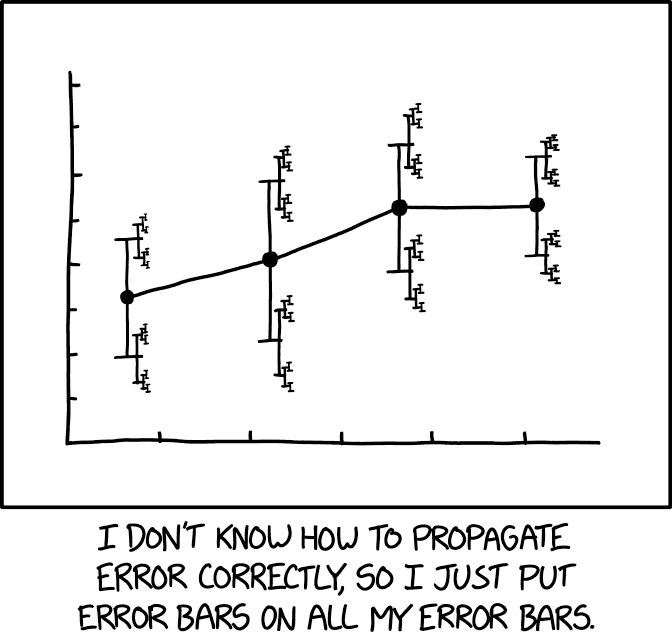156

8

15

8

# Measurements.jl

## Introduction

### What Is This Package Useful For?Image credit: "xkcd: Error Bars" (CC-BY-NC 2.5)

Measurements.jl relieves you from the hassle of propagating uncertainties coming from physical measurements, when performing mathematical operations involving them. The linear error propagation theory is employed to propagate the errors.

This library is written in Julia, a modern high-level, high-performance dynamic programming language designed for technical computing.

When used in the Julia interactive session, it can serve also as an easy-to-use calculator.

### Features List

• Support for most mathematical operations available in Julia standard library and special functions from SpecialFunctions.jl package, involving real and complex numbers. All existing functions that accept AbstractFloat (and Complex{AbstractFloat} as well) arguments and internally use already supported functions can in turn perform calculations involving numbers with uncertainties without being redefined. This greatly enhances the power of Measurements.jl without effort for the users
• Functional correlation between variables is correctly handled, so x-x ≈ zero(x), x/x ≈ one(x), tan(x) ≈ sin(x)/cos(x), cis(x) ≈ exp(im*x), etc...
• Support for arbitrary precision (also called multiple precision) numbers with uncertainties. This is useful for measurements with very low relative error
• Define arrays of measurements and perform calculations with them. Some linear algebra functions work out-of-the-box
• Propagate uncertainty for any function of real arguments (including functions based on C/Fortran calls), using @uncertain macro
• Function to get the derivative and the gradient of an expression with respect to one or more independent measurements
• Functions to calculate standard score and weighted mean
• Parse strings to create measurement objects
• Easy way to attach the uncertainty to a number using the ± sign as infix operator. This syntactic sugar makes the code more readable and visually appealing
• Extensible in combination with external packages: you can propagate errors of measurements with their physical units, perform numerical integration with QuadGK.jl, numerical and automatic differentiation, and much more.
• Integration with Plots.jl.

The method used to handle functional correlation is described in this paper:

A current limitation of the package is that it is not yet possible to define quantities related by a correlation matrix.

If you use use this package for your research, please cite it.

### Documentation

The complete manual of Measurements.jl is available at https://juliaphysics.github.io/Measurements.jl/stable/. There, people interested in the details of the package, in order integrate the package in their workflow, can can find a technical appendix explaining how the package internally works.

## Installation

The latest version of Measurements.jl is available for Julia v1.0 and later releases, and can be installed with Julia built-in package manager. In a Julia session, after entering the package manager mode with ], run the command

pkg> update


Older versions of this package are also available for Julia 0.4-0.7.

## Usage

After installing the package, you can start using it with

using Measurements


The module defines a new Measurement data type. Measurement objects can be created with the two following constructors:

measurement(value, uncertainty)
value ± uncertainty


where

• value is the nominal value of the measurement
• uncertainty is its uncertainty, assumed to be a standard deviation.

They are both subtype of AbstractFloat. Some keyboard layouts provide an easy way to type the ± sign, if your does not, remember you can insert it in Julia REPL with \pm followed by TAB key. You can provide value and uncertainty of any subtype of Real that can be converted to AbstractFloat. Thus, measurement(42, 33//12) and pi ± 0.1 are valid.

measurement(value) creates a Measurement object with zero uncertainty, like mathematical constants. See below for further examples.

Every time you use one of the constructors above, you define a new independent measurement. Instead, when you perform mathematical operations involving Measurement objects you create a quantity that is not independent, but rather depends on really independent measurements.

Most mathematical operations are instructed, by operator overloading, to accept Measurement type, and uncertainty is calculated exactly using analityc expressions of functions’ derivatives.

In addition, it is possible to create a Complex measurement with complex(measurement(a, b), measurement(c, d)).

measurement(string)


measurement function has also a method that enables you to create a Measurement object from a string.

This module extends many methods defined in Julia’s mathematical standard library, and some methods from widespread third-party packages as well. This is the case for most special functions in SpecialFunctions.jl package, and the quadgk integration routine from QuadGK.jl package. See the full manual for details.

## Examples

julia> using Measurements

julia> a = measurement(4.5, 0.1)
4.5 ± 0.1

julia> b = 3.8 ± 0.4
3.8 ± 0.4

julia> 2a + b
12.8 ± 0.4472135954999579

julia> x = 8.4 ± 0.7

julia> x - x
0.0 ± 0.0

julia> x/x
1.0 ± 0.0

julia> x*x*x - x^3
0.0 ± 0.0

julia> sin(x)/cos(x) - tan(x)
-2.220446049250313e-16 ± 0.0 # They are equal within numerical accuracy


The Measurements.jl package is licensed under the MIT "Expat" License. The original author is Mosè Giordano.

Please, cite the paper Giordano 2016 (http://arxiv.org/abs/1610.08716) if you employ this package in your research work. For your convience, a BibTeX entry is provided in the CITATION.bib file.

05/17/2016

1 day ago

356 commits